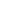# Relate doubles to even numbers, and write number sentences to express the sums

EngageNY 60 min(s)
In this lesson, students arrange doubles into rectangular arrays (e.g., 2 rows of 7, or 2 sevens) and write an equation to show the total as a sum of two equal addends (e.g., 7 + 7 = 14). They discover that doubles yield even numbers even when the number being doubled is not even.